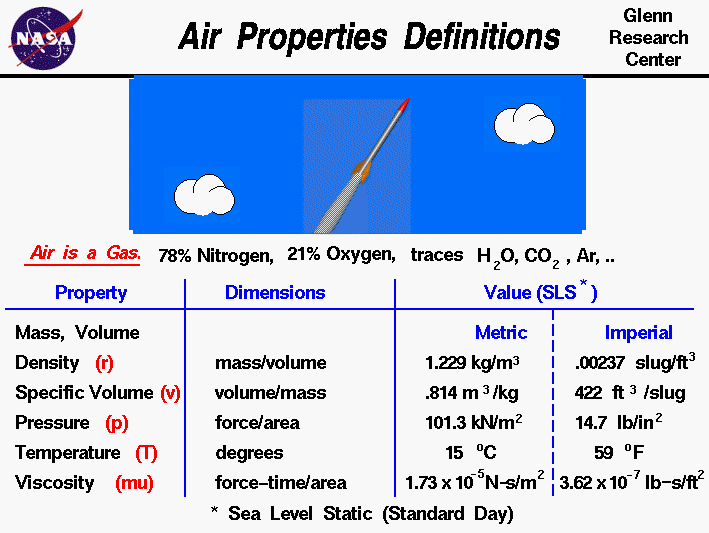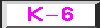+ Text Only Site
+ Non-Flash Version
+ Contact GlennThis page is intended for college, high school, or middle school students. For younger students, a simpler explanation of the information on this page is available on the Kids Page.Earth's atmosphere is composed of air. Air is a mixture of gases, 78% nitrogen and 21% oxygen with traces of water vapor, carbon dioxide, argon, and various other components. We usually model air as a uniform (no variation or fluctuation) gas with properties that are averaged from all the individual components. Any gas has certain properties that we can detect with our senses. The values and relations of the properties define the state of the gas.

On this slide you will find typical values of the properties of air at sea level static conditions for a standard day. We are all aware that pressure and temperature of the air depend on your location on the earth and the season of the year. And while it is hotter in some seasons than others, pressure and temperature change day to day, hour to hour, sometimes even minute to minute during severe weather. The values presented on the slide are simply average values used by engineers to design machines. That's why they are called standard values. We also know that all of the state-of-the-gas variables will change with altitude, which is why the typical values are given at sea level, static conditions. Because the gravity of the Earth holds the atmosphere to the surface, as altitude increases, air density, pressure, and temperature (for lower altitudes) decrease. At the edge of space, the density is almost zero. The variation of the air from the standard can be very important since it affects flow parameters like the speed of sound.

A gas is composed of a large number of molecules which are in constant, random motion. The sum of the mass of all the molecules is equal to the mass of the gas. A gas occupies some volume in three dimensional space. For a given pressure and temperature, the volume depends directly on the amount of gas. Since the mass and volume are directly related, we can express both the mass and volume by a single variable. When a gas is moving, it is convenient to use the density of a gas, which is the mass divided by the volume the gas occupies. The sea level standard value of air density r is

r = 1.229 kilograms/cubic meters = .00237 slug/cubic feet

When working with a static or unmoving gas, it is more convenient to use specific volume, which is the volume divided by the mass. The sea level standard value of specific volume v is

v = .814 cubic meters/kilogram = 422 cubic feet/slug

The pressure of a gas equals the perpendicular force exerted by the gas divided by the surface area on which the force is exerted. The sea level standard value of air pressure p is

p = 101.3 kilo Newtons/square meter = 14.7 pounds/square inch

The temperature of a gas is a measure of the kinetic energy of the molecules of the gas. The sea level standard value of air temperature T is

T = 15 degrees C = 59 degrees Fahrenheit

A gas can exert a tangential (shearing) force on a surface, which acts like friction between solid surfaces. This "sticky" property of the gas is called the viscosity and it plays a large role in aerodynamic drag. The sea level standard value of air viscosity mu is

mu = 1.73 x 10^-5 Newton-second/square meters = 3.62 x 10^-7 pound-second/square feet

The density (specific volume), pressure, and temperature of a gas are related to each other through the equation of state. The state of a gas can be changed by external processes, and the reaction of the gas can be predicted using the laws of thermodynamics. A fundamental understanding of thermodynamics is very important in describing the operation of propulsion systems.

Activities:Guided Tours+ Inspector General Hotline + Equal Employment Opportunity Data Posted Pursuant to the No Fear Act + Budgets, Strategic Plans and Accountability Reports + Freedom of Information Act + The President's Management Agenda + NASA Privacy Statement, Disclaimer, and Accessibility CertificationEditor: Nancy Hall NASA Official: Nancy Hall Last Updated: May 13 2021 + Contact Glenn